Math Concepts
Two-way Tables and Frequency Tables
8.3k views

 1 Introduction 2 Types of Data 3 Introduction to Graphical Data 4 Two-way frequency tables 5 Making two-way tables 6 Two-way relative frequency table 7 Conclusion 8 FAQs

18 December  2020

Reading Time: 6 Minutes

## Introduction

A two-way table is a way to display frequencies or relative frequencies for two variables.

One of the variables is represented using rows and the other one using columns.

They are used to see if there is any relationship between the two variables.

Here are some additional points that talk about how to two-way tables. To view them click on the Download button.

 📥 Two-way tables and frequency tables Download

## Types of Data

### Discrete Data

• It can only take certain values.
• It can be counted.
• It cannot be divided.
• It can be graphed on a bar graph.
• Example: number of dogs, number of books, number of students etc.

### Continuous Data

• It can take any value within a range.
• It can be measured.
• It can be divided.
• It can be graphed on a histogram or a line graph.
• Example: time, the weight of a dog, the height of the students etc.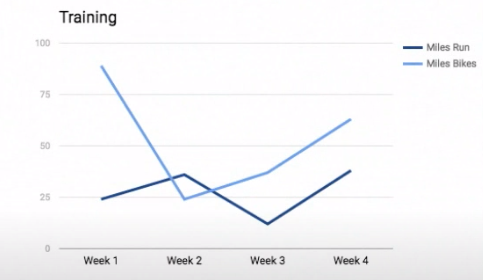## Introduction to Graphical Data

Various charts and graphs can be used to visually display and analyze the graphical data.

It helps us to quickly highlight the behavior and relationship between data points which may contribute to better understanding and predictions.

Depending on the type of data we may use a bar graph, histogram, line graph, heat map, Venn diagram, pie chart, etc.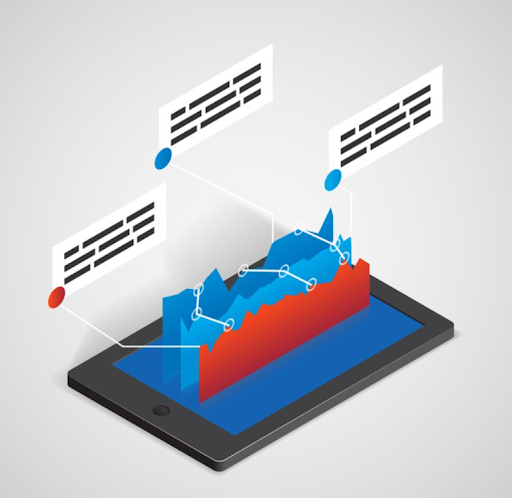## Two-way frequency tables

The two-way frequency table shows how many data points fit into each category.

It’s a good way to organize data based on two categorical variables.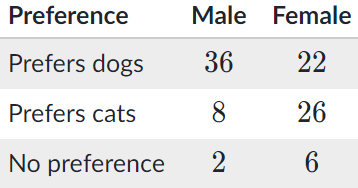## Making two-way tables

1. Identify the two variables of interest.
2. Determine the possible values of each variable.
3. Pick one variable to be represented by the rows and the other to be represented by the columns.
4. Fill the table with frequencies.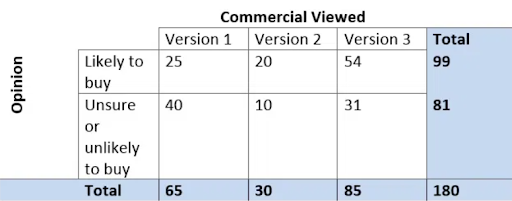## The Two-way relative frequency table

The two-way relative frequency table uses relative frequencies like percentages or proportions instead of the frequency counts. It shows what percentage of the data points fit in each category.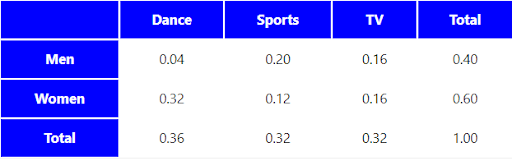## Conclusion

Two-way tables and frequency tables help us to represent the comparison of two variables.

This can be used in data visualization and data analysis to arrive at important decisions.

A good understanding of these also improves basic aptitude skills and logical reasoning skills.

Cuemath, student-friendly mathematics and coding platform, conducts regular Online Live Classes for academics and skill-development, and their Mental Math App, on both iOS and Android, is a one-stop solution for kids to develop multiple skills.

Check out the fee structure for all grades and book a trial class today!

## What is data?

Data is a collection of facts and statistics for analysis.

## How do you differentiate between data and information?

Data is a collection of facts. Whereas, information is how you understand those facts.

## What are the ways to represent data?

Data can be represented using graphs, tables and charts.

## What are the different types of graphs?

Various types of graphs are bar graph, line graph, pictograph etc.

## What is a two-way table?

Two-way table displays relative frequencies for two categorical variables.

## When is a two-way table used?

Two-way table is useful when there are different sample sizes in dataset.

Related Articles